# Properties of Solids and Liquids   Share

## What is Properties of Solids and Liquids

As you know solid, liquid, gas is the most observable state of matter in our everyday life. Properties of solids, liquids, and gases are different from each other. Properties of Solids and Liquids chapter will help you to understand the important properties of solids and liquids. Because learning properties about Solids and Liquids will also help us in everyday life.

Have you seen a beam balance in any shop?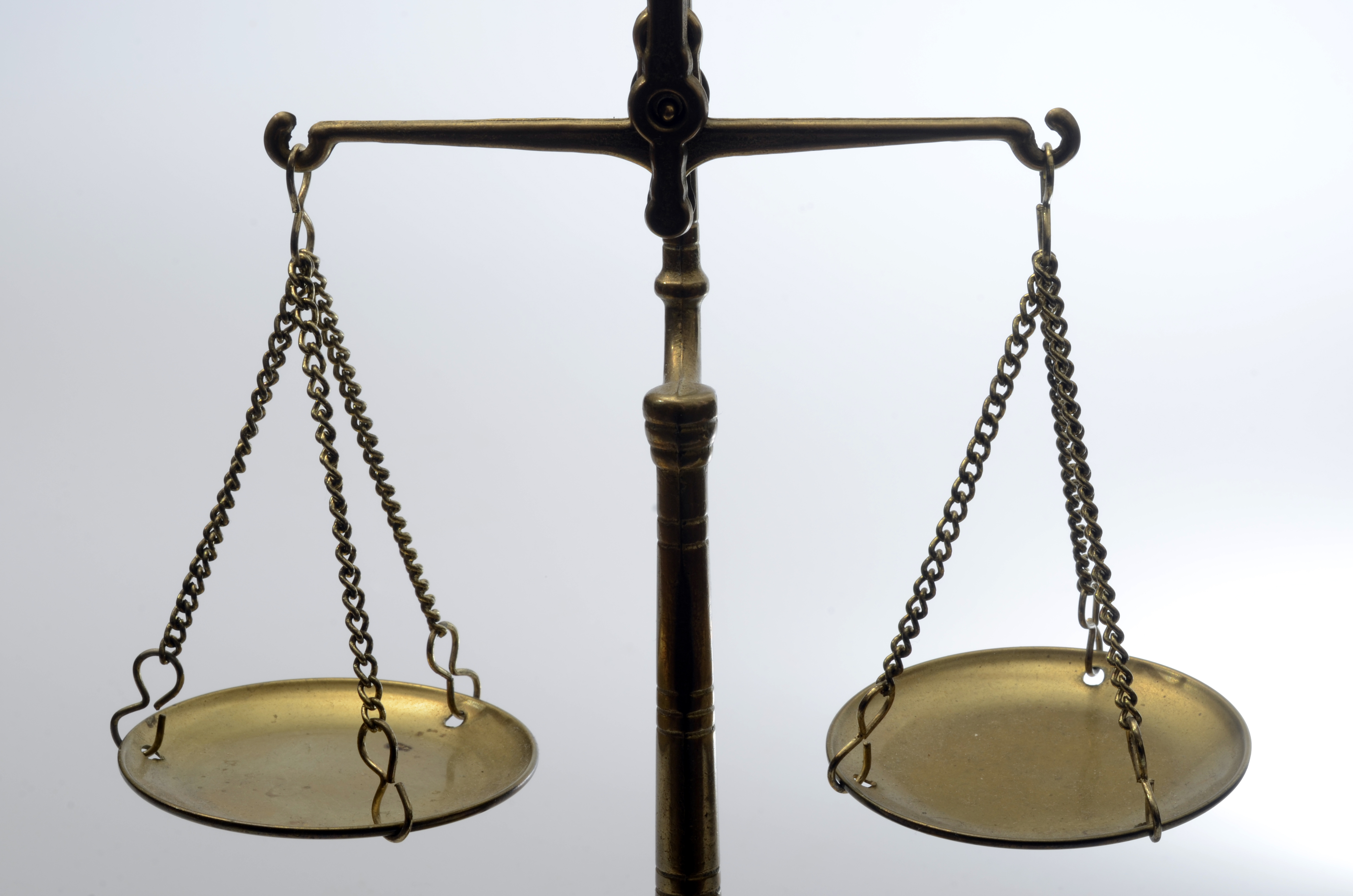So in a beam balance if you have to weigh something on left pan then you have to put equal weight on the right pan. But what if we have to weigh a big car or big truck. Either you can take a very big beam balance with very big pans, and then take a reading of its weight, which seems very difficult or not practically applicable. But if we learn some basic properties about fluid and modified our measuring instrument then we can measure big cars weights easily. This can be done with the help of Hydraulic Lift. In this device, are two pistons which are separated by the space filled with a liquid. And using the concept of same pressure on the same horizontal line we are able to do this.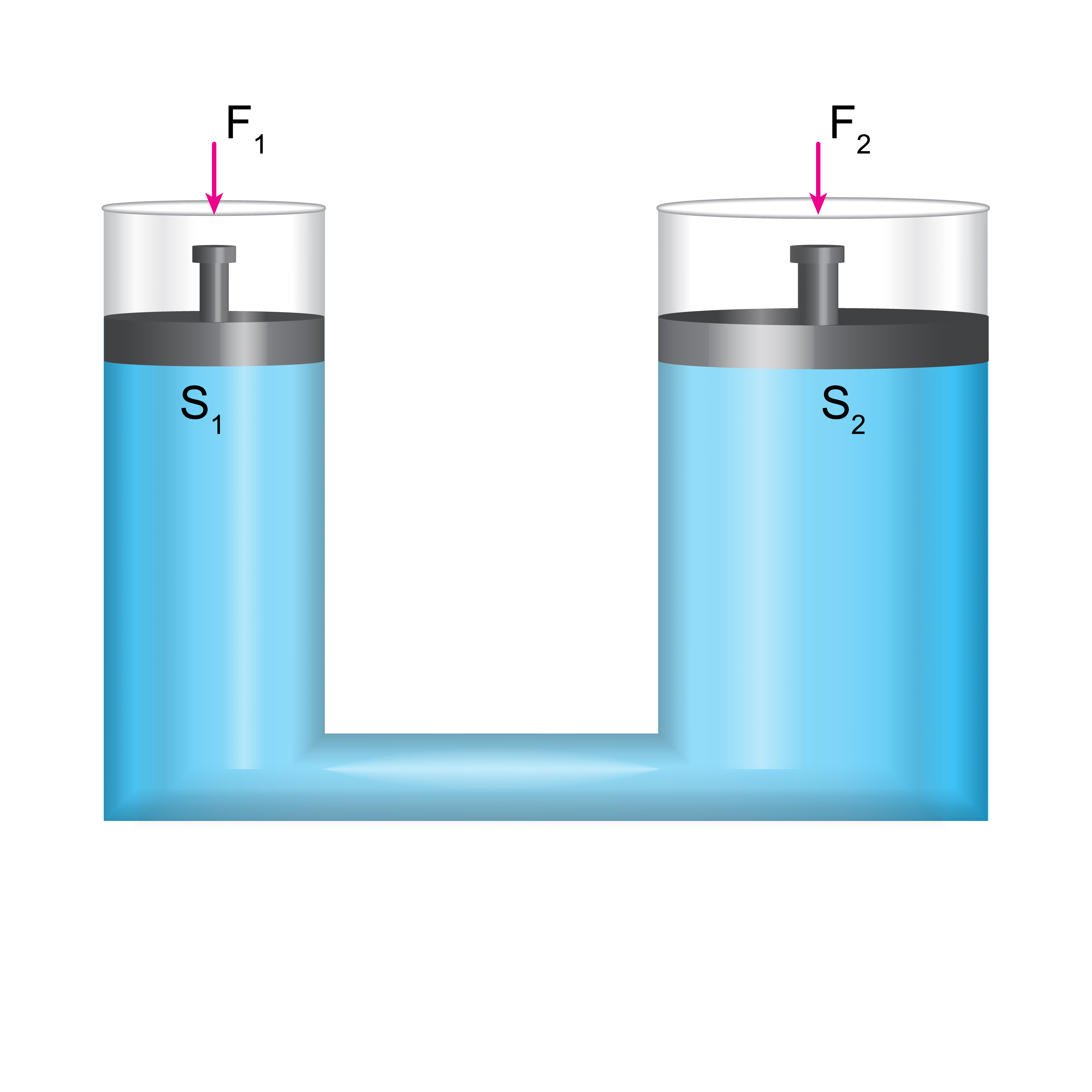As shown in figure both the piston are at the same horizontal level. So they will have the same pressure.

Which gives us

SO keeping

We will get

So small force (  ) can be able to balance big wight cars, truck choosing suitable areas of the piston ( i.e  )

In a similar way, we will learn various mechanical and thermal properties of solids and liquids in this chapter. We will also learn the importance of these properties. Properties of Solids and Liquids chapter is one of the most important chapters while preparing for all competitive exam. The Concept of Properties of Solids and Liquids and other chapters of mechanics and thermodynamics are mixed in miscellaneous questions which are asked in competitive exams.

Prepare Online for JEE Main/NEET

Crack JEE 2021 with JEE/NEET Online Preparation Program

## Notes on Properties of Solids and Liquids-

So we will discuss step by step about important topics from this chapter followed by an overview of this chapter.

Then we will understand important formulas from this chapter. Remembering these formulas will increase your speed while question-solving.

## Properties of Solids and Liquids  Topics

• Elastic behavior
• Stress and strain relationship, Hooke's Law
• Young's modulus, bulk modulus, modulus of rigidity.
• Pressure, Pascal's law, and its applications.
• Viscosity, terminal velocity, Stokes' law
• streamline and turbulent flow, Reynolds number
• Bernoulli's principle and its applications.
• Surface energy and surface tension and its applications, angle of contact, capillary rise.
• Heat and temperature,
• Thermal expansion of solids, liquids
• Specific heat capacity, calorimetry; change of state, change of phase, latent heat.
• Heat transfer and its types like conduction, convection, and radiation, Newton's law of cooling.

## Properties of Solids and Liquids Overview

In this chapter,

• You will learn two important topics of Mechanical Properties of Solids which are stress and strain. If you understand these topics then it would be easy for you to understand all the modulus of elasticity, comparing the restoring force of wire, etc.
• You will learn about liquids and its important properties like pressure, Viscosity, Surface energy, and surface tension and with the help of concepts like Pascal's Law,  Stokes' law, Bernoulli's Principle you will able to understand these properties easily. Working of Capillary, Venturimeter, Hydraulic Lift are based on applications of these laws.
• Bernoulli's Principle is a very important concept in this chapter. When a spinning ball is thrown it deviates from its usual path in flight, During a tornado or hurricane Blowing off roofs by wind storms, Working of an airplane all can be understood with the help of  Bernoulli's application.
• Viscosity is also an important concept from this chapter, which is also known as fluid friction or internal friction.
• After studying mechanical properties you will learn about important thermal properties of Matter like thermal expansion, Thermal Stress, and strain, specific heat capacity, latent heat along with the importance of these properties.
• You will learn about temperature which is the degree of hotness or coldness of a body.
• Then you will learn about Thermal expansion because of which a matter can change its shape, area, and volume when there is a change in temperature. You will also learn the effect of Thermal expansion on a matter like there is Loss of time in a time period of Simple Pendulum due to increase in temperature. Thermal Stress and Thermal Strain are produced in the material due to this Thermal Expansion.
• Then you will learn about heat and different Heat transfer methods like conduction, convection, and radiation. You will also learn about Newton's Law of Cooling, Stefan's Law which will help you to understand the heat transfer.

## Properties of Solids and Liquids Formulas

1.

2. Longitudinal strain=

3. Volumetric strain=

4. Shearing strain=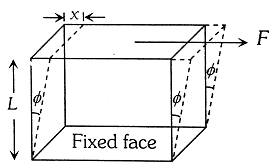5. Stress vs strain curve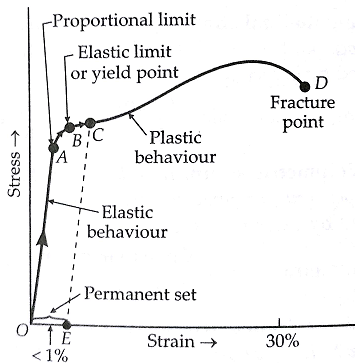1. HOOKE's LAW=

2. Young Modulus==

3. Modulus of Rigidity (Shear modulus)==

4. Energy Stored per unit Volume=

5. Pressure=

6. Viscous Force=

7. Terminal Velocity=

8. Bernoulli's Principle-

9. Surface Tension=

10. Ascent Formula=

11. Coefficient of Linear Expansion=

12. Specific Heat=

13. Heat Current=

14. Newton's Law of Cooling=

## How to prepare Properties of Solids and Liquids

• First, you should have good command over the concepts of the Properties of Solids and Liquids and you should also know how to apply them well at the time of the entrance exam or while solving questions.
• Please try to understand each concept from this chapter, with the help of theory, questions with solutions and video lectures on each important concept.
• For each concept practice enough problems to have a thorough understanding of the concept. Solve all the questions at home with proper concentration and try to do all calculations by yourself without seeing the solution first.
• Formulae for spring can be used for wire also using spring constant for wire as  .
• Topics like stress and strain are interrelated so study both the topics.
• Practice lots of questions based on the concept of Bernoulli's Principle. Surface Energy and Surface Tension are also important concepts from this chapter. A lot of questions are asked to find out Excess Pressure or Change in Pressure of bubble in air/liquid.
• Also, Understand the concept of Thermal expansion and its effects on matter. Remember the formulas from this chapter because in certain questions you will get the answer by direct applying these formulas.
• Understand all the laws from this chapter along with their applications. With proper study, you can solve questions from this chapter with ease.

## Properties of Solids and Liquids Tips

1. Make a plan to prepare for this chapter and Stick to a Timetable.

2. Plan a daily schedule for study, And have a fresh start each day to improve your preparation each day.3. Study the concept first and then start solving the question. Don't go through question directly without knowing the concept.

4. Solve previous year question from this chapter.

5. Use smart methods to solve questions.

## Properties of Solids and Liquids Books

For Properties of Solids and Liquids, chapter concepts in NCERT are enough but you will have to practice lots of questions including previous year questions and you can follow other standard books available for competitive exam preparation like Concepts of Physics (H. C. Verma) and Understanding Physics by D. C. Pandey (Arihant Publications).

## Physics Chapter wise Notes For Engineering and Medical Exams

 Chapters No. Chapters Name Chapter 1 Physics and Measurement Chapter 2 Kinematics Chapter 3 Laws of motion Chapter 4 Work Energy and Power Chapter 5 Rotational Motion Chapter 6 Gravitation Chapter 8 Kinetic theory of Gases Chapter 9 Thermodynamics Chapter 10 Oscillations and Waves Chapter 11 Electrostatics Chapter 12 Current Electricity Chapter 13 Magnetic Effects of Current and Magnetism Chapter 14 Electromagnetic Induction and Alternating currents Chapter 15 Electromagnetic Waves Chapter 16 Optics Chapter 17 Dual Nature of Matter and Radiation Chapter  18 Atoms And Nuclei Chapter 19 Electronic devices Chapter 20 Communication Systems Chapter 21 Experimental skills

### Topics from Properties of Solids and Liquids

• Pressure due to fluid column ( JEE Main, COMEDK UGET ) (20 concepts)
• Pascal's law and its applications ( JEE Main, COMEDK UGET ) (5 concepts)
• Specific heat capacity, Calorimetry, Change os state and latent heat ( JEE Main, COMEDK UGET ) (29 concepts)
• Streamline, Turbulent flow and Reynold'snumber ( JEE Main, COMEDK UGET ) (9 concepts)
• Viscosity, Stoke's law, Terminal velocity ( JEE Main, COMEDK UGET ) (16 concepts)
• Bernoulli's principle and its applications ( JEE Main, COMEDK UGET ) (6 concepts)
• Surface energy and surface tensions ( JEE Main, COMEDK UGET ) (16 concepts)
• Elastic behaviour ( JEE Main, COMEDK UGET ) (10 concepts)
• Stress-strain relationship ( JEE Main, COMEDK UGET ) (17 concepts)
• Hooke's law ( JEE Main, COMEDK UGET ) (1 concepts)
• Young modulus and bulk modulus and modulus of rigidity ( JEE Main, COMEDK UGET ) (18 concepts)
• Heat, temperature, thermal expansion ( JEE Main, COMEDK UGET ) (49 concepts)
• Heat transfer and its types ( JEE Main, COMEDK UGET ) (59 concepts)
• Newton's law of cooling ( JEE Main, COMEDK UGET ) (8 concepts)
• Fluid dynamics ( JEE Main, COMEDK UGET ) (8 concepts)
• Surface Tension ( JEE Main, COMEDK UGET ) (5 concepts)
• Thermal properties of matter ( JEE Main, COMEDK UGET ) (6 concepts)
• Heat transfer ( JEE Main, COMEDK UGET ) (21 concepts)
• Properties of solids ( JEE Main, COMEDK UGET ) (7 concepts)
• Fluid statics ( JEE Main, COMEDK UGET ) (6 concepts)

### Important Books for Properties of Solids and Liquids

•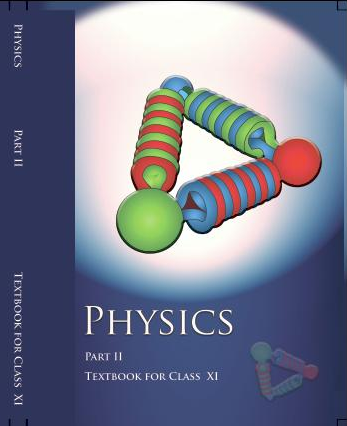•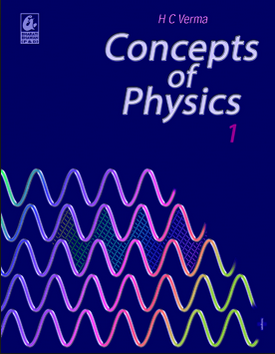•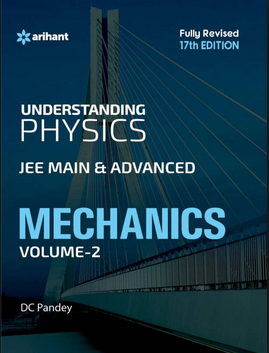Exams
Articles
Questions